# Stars

Relevant articles: , .

[hide]

# Stars

The star on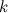$k$ vertices, denoted by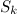$S_k$, consists of a vertex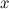$x$ of degree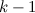$k-1$, its$k-1$ neighbours, and the$k-1$ edges joining$x$ to its neighbours. Note that stars are trees. Also note that a star$S_k$ is the complete bipartite graph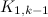$K_{1,k-1}$.

## Spectrum Results

The spectrum problem for stars was completely settled by Yamamoto et al in  and independently by Tarsi in .

Theorem 1 

Let$k\geq 2$. There exists an$S_k$-design of order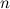$n$ if and only if

•$n=1$ or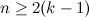$n \geq 2(k-1)$; and
•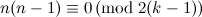$n(n-1)\equiv 0 \,({\rm mod \ }2(k-1))$.

## Notes

• Sometimes$S_k$ is used to denote a star with$k$ edges, rather than$k$ vertices, for example in .

## References

1. 1.0 1.1 Tarsi, M. Decomposition of complete multigraphs into stars, Discrete Math. 26, 273–278 (1979).
2. 2.0 2.1 2.2 Yamamoto, S., Ikeda, H., Shige-eda, S., Ushio, K., and Hamada, N. On claw-decomposition of complete graphs and complete bigraphs, Hiroshima Math. J. 5, 33–42 (1975).
3. Adams, P., Bryant, D., and Buchanan, M. A survey on the existence of G-designs, J. Combin. Des. 16, 373–410 (2008).